# Test Prep Plan - Take a practice test

Take this practice test to check your existing knowledge of the course material. We'll review your answers and create a Test Prep Plan for you based on your results.
How Test Prep Plans work
1
2Based on your results, we'll create a customized Test Prep Plan just for you!
3Study smarter
Study more effectively: skip concepts you already know and focus on what you still need to learn.

# NES Math: Area Under the Curve & Integrals Chapter Exam

Exam Instructions:

Choose your answers to the questions and click 'Next' to see the next set of questions. You can skip questions if you would like and come back to them later with the yellow "Go To First Skipped Question" button. When you have completed the practice exam, a green submit button will appear. Click it to see your results. Good luck!

### Page 1

#### Question 1 1. Which of the following sums represents an n-term Riemann sum for the integral below?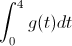#### Question 2 2. What is the one term left-sided Riemann Sum approximation for the integral below?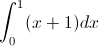#### Question 3 3. Use the average value theorem to determine the average value of f(x) between 0 and 2.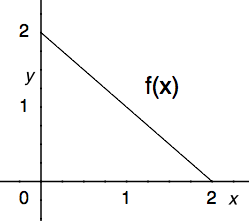#### Question 4 4. Which of the following integrals represents the area under the graph below?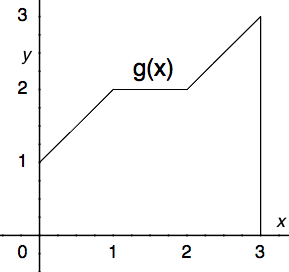#### Question 5 5. What is the two term left-sided Riemann Sum approximation for the integral below?### Page 2

#### Question 6 6. Given the following information, what does z equal?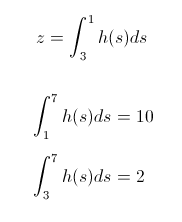#### Question 7 7. Using the single term Riemann sum as shown, what is the area beneath the curve f(x) between 0 and two pi?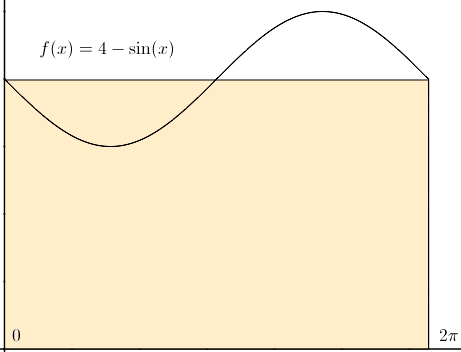#### Question 8 8. Which of the following shows the correct method for evaluating the integral below?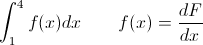#### Question 9 9. Evaluate the following.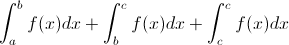#### Question 10 10. Use the fundamental theorem of calculus to solve the problem below.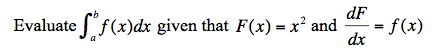### Page 3

#### Question 11 11. Which of the following graphs shows the correct region of integration for the integral below?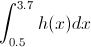#### Question 12 12. Which of the following represents the area of the FIRST rectangle being used to estimate the area under the curve f(x) between x = 0 and x = 2 when divided into 4 slices?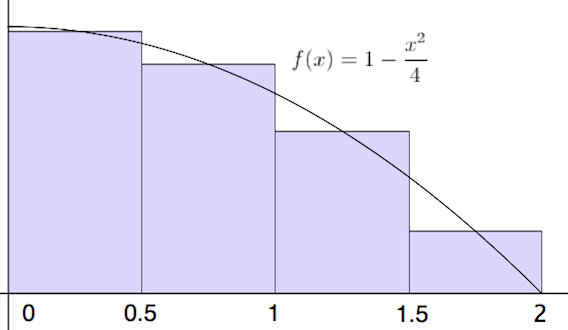#### Question 13 13. Given f(x) on the graph below, which of the following integrals will equal 0?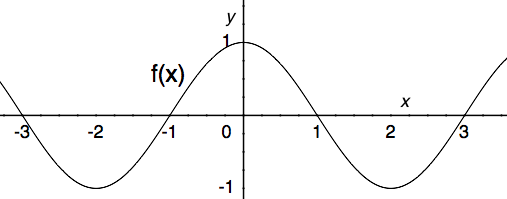#### Question 14 14. Evaluate the following integral.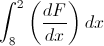#### Question 15 15. In the following equation, v(t) is the _____.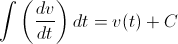### Page 4

#### Question 16 16. Which integral would be evaluated using the following expression (given that F'(x) = f(x))?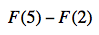#### Question 18 18. Estimate the area beneath the curve f(x) using the two term Riemann sum as shown below.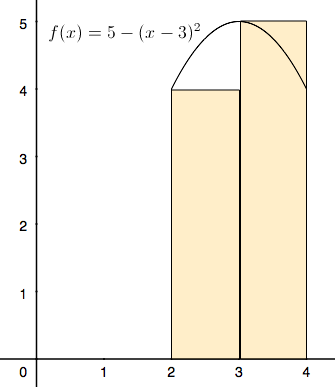#### Question 19 19. The following integral can be re-written as: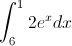#### Question 20 20. How many terms are in the Riemann sum represented below?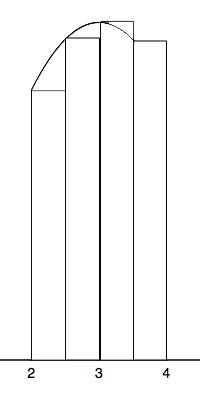### Page 5

#### Question 21 21. Given f(x) on the graph, what does z equal?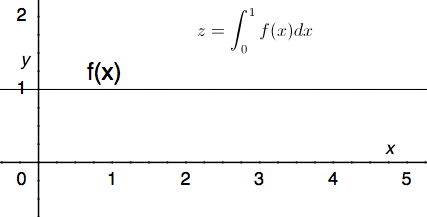#### Question 23 23. You are going to take a Riemann Sum of the area below. To do this, you divide the region into four slices of equal width. How wide is each slice?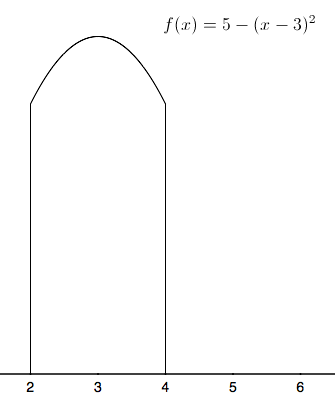#### Question 24 24. Use the fundamental theorem of calculus to evaluate the following integral.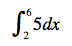### Page 6

#### Question 26 26. The average value for this continuous function over the shaded region is 2. According to the average value theorem, how many times does this function pass through the value 2?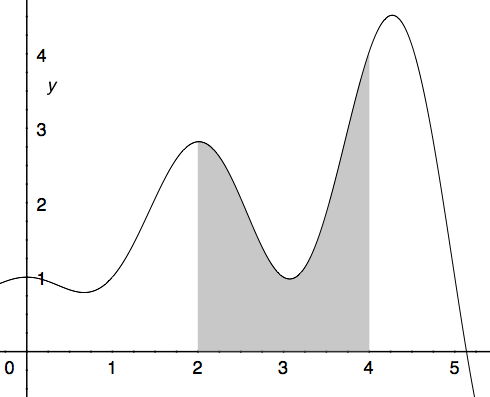#### Question 27 27. In the following equation, g(s) is the _____.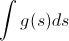#### Question 28 28. Which integral below is equal to the following integral?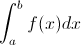#### Question 29 29. Which of the following represents a left-sided Riemann sum of the following integral with n = 4?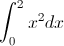#### Question 30 30. Which of the following expressions is equal to the integral below?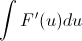#### NES Math: Area Under the Curve & Integrals Chapter Exam Instructions

Choose your answers to the questions and click 'Next' to see the next set of questions. You can skip questions if you would like and come back to them later with the yellow "Go To First Skipped Question" button. When you have completed the practice exam, a green submit button will appear. Click it to see your results. Good luck!

Support